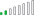cancel
Showing results for
Did you mean:Anonymous
Not applicable

## calculate number of working days between 2 dates

We have a table with tasks:

We also have a table with all days in the year, indicating the date as well as attributes like day of the week

And we have a table with the holidays in the year.

We would like to calculate the number of days between End and Start date for each task. We know that we can subtract those 2 dates to get a number, but we woudl like to exclude weekends and holidays. Is there any way to do that in Power BI Desktop?

1 ACCEPTED SOLUTIONMicrosoft

@Anonymous

With tables as below, you can either use a calculated column or a measure.```Total Working Days Column =
SUMX (
FILTER (
'Calendar',
),
'Calendar'[isWorkDay]
)

Total Working Days Measure =
SUMX (
FILTER (
'Calendar',
),
'Calendar'[isWorkDay]
)```
5 REPLIES 5Microsoft

@Anonymous

With tables as below, you can either use a calculated column or a measure.```Total Working Days Column =
SUMX (
FILTER (
'Calendar',
),
'Calendar'[isWorkDay]
)

Total Working Days Measure =
SUMX (
FILTER (
'Calendar',
),
'Calendar'[isWorkDay]
)```Frequent Visitor

Hi Eric

This works great in the scenario where StartDate is always before EndDate... How to ensure that it still calculates correctly in cases where StartDate is after EndDate?
- currently it only gives me blank values for all of these (which makes sense), but what I would like to measure this same distance, but in the opposite direction?Resolver II

Hey @Eric_Zhang

I tried your solution as a calulated column ( I already had a date dimension build in my report so re-used it).

For some reason when I use your solution I'm getting 1 where I'd expect a 0 when start date and finish date happen to be on the same day? I might be wrong?I'm using your solution to check how many working days an activity is early or late by and in above snapshot, if you look at a seconds line for example, activity was completed on time but I get 1 indicating it's a day late.

Any suggestions for this kind of issue?

ThanksAnonymous
Not applicable

Probably easiest to add a calc column to your date table IsWorkDay (which uses LOOKUPVALUE into your holidays table and Sat/Sun sorta thing).

Here is a totally un-tested measure that should probably work for you:

```Total Working Days = CALCULATE (
COUNTROWS(Calendar),
Calendar[IsWorkDay] = TRUE( ),
)```Solution Sage

Hi @Anonymous. I was trying to figure this one out too, and tested your measure for my own learning. It got me in the right direction, but it needs a FILTER. As is:@Anonymous. With just a little modification, you'll get the result you want:

```Total Working Days = CALCULATE(
COUNTROWS('Calendar'),
Calendar[IsWorkday] = TRUE(),
)
)```

FYI: When using Calendar as a name for a table, it looks like you have to wrap it in single quotes when using it without a column reference.

If the IsWorkday column doesn't make sense, it should look something like the photo below, where there is a relationship between the Calendar table and Holidays table based on the Date.